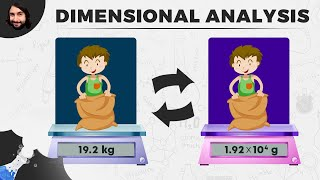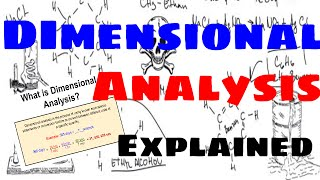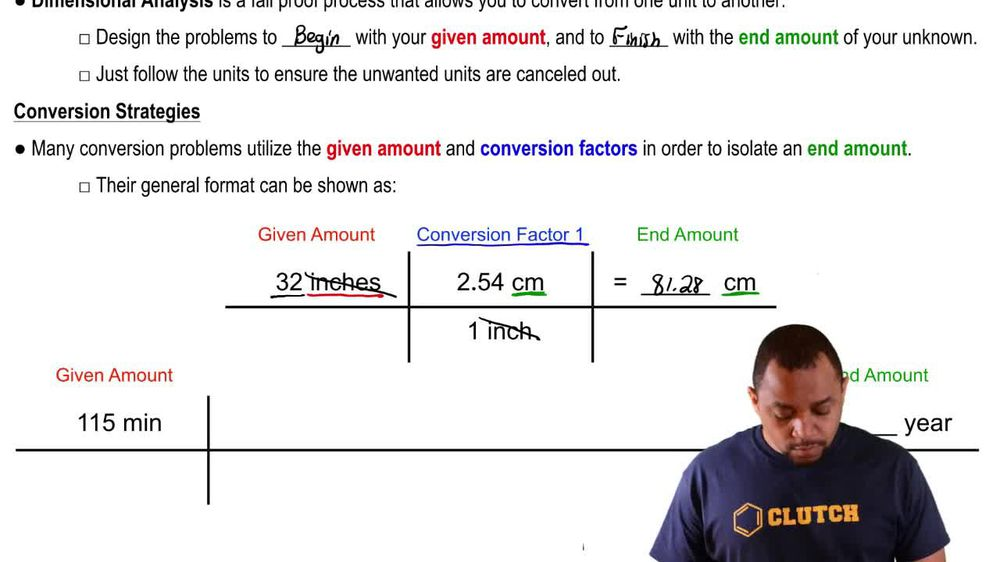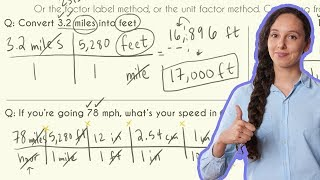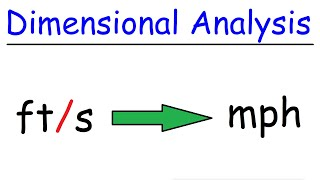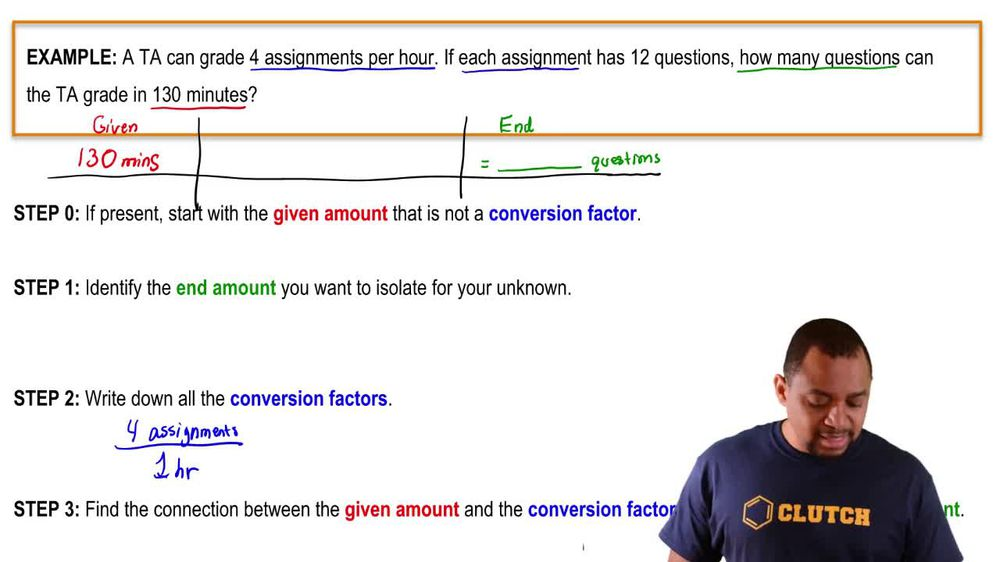Start typing, then use the up and down arrows to select an option from the list.
1. 1. Intro to General Chemistry2. Dimensional Analysis
Problem

# Perform each unit conversion. a. 1.4 in to mm b. 116 ft to cm c. 1845 kg to lb d. 815 yd to km

Relevant Solution2m
Play a video:
Hello, everyone in this video, we're going to convert miles into centimeters. So what we're doing here is called the metro analysis. Alright. So to go from miles into centimeters, what is the direct conversion? I'm not sure if you know, but I definitely do not. So I'm gonna go ahead and do baby steps and convert everything into maybe units that I do know. So my game plan, my game plan or road map, I'm gonna go ahead and start off with the miles because that's what I'm given, I'm gonna convert that into kilometers and then into meters And lastly two cm. So these are the units that I've chosen because I know the direct conversions from each of those, but you can go ahead and maybe do even more or less, but this is just my game plan. Alright, so starting off with the one .49 mi and I'm given, I'm gonna go ahead and convert the miles into kilometers. So I'm starting here going to here. Okay, so For every one km that's going to be 0.6 to 14 miles, then I want to go ahead and go from my kilometers into my meters. So I know every one kilometer There's going to be 1000 m not going from meters into centimeters, which is my final destination. I know that every one centimeter it's a one or 0.1 meter, So you can see here that the units of miles will cancel units of kilometers will cancel and units of meter, will cancel leaving us with just 3cm as desired. Alright, so putting all of this into my calculator, I will end up with the value of 2. times 10 to the 5th power cm and that's going to be my final answer for this problem.